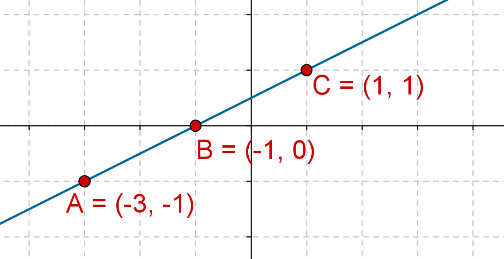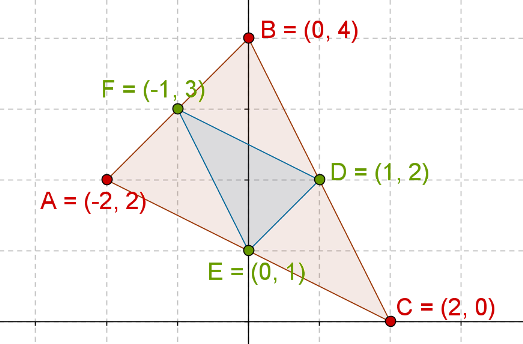# Collinearity

Go back to  'Coordinate-Geometry'

Consider the following three points:

\begin{align}&A\left( { - 3,\; - 1} \right)\\&B\left( { - 1,\;0} \right)\\&C\left( {1,\;1} \right)\end{align}

If we plot these points in the plane, we find that they are collinear:Now, let us apply the formula for the area of a triangle on these three points:

\begin{align}&{\rm{Area}}\left( {\Delta ABC} \right) = \frac{1}{2}\;\left| {\,\begin{array}{*{20}{c}}{ - 3}&{ - 1}&1\\{ - 1}&0&1\\1&1&1\end{array}\;} \right|\\&= \frac{1}{2}\;\left| { - 3\left( {0 - 1} \right) + \left( { - 1} \right)\left( {1 - \left( { - 1} \right)} \right) + 1\left( { - 1 - 0} \right)} \right|\\&= \frac{1}{2}\;\left| {3 - 2 - 1} \right|\\& = \frac{1}{2} \times 0\\&= 0\;{\rm{sq}}{\rm{.}}\;{\rm{units}}\end{align}

The answer is not surprising. Since A, B and C are collinear, the “triangle” formed by these three points will obviously have zero area.

This can be used as an effective test for collinearity of three points. If the area of the triangle formed by any three distinct points is zero, then those three points must be collinear. For any three non-collinear points, the area must always be non-zero, which means that the only case where three distinct points can give rise to a triangle with zero area is when the three points are collinear.

Example 1: Consider the following three points:

\begin{align}&A\left( {2,\;3} \right)\\&B\left( {6,\; - 3} \right)\\&C\left( {4,\;k} \right)\end{align}

If these points are collinear, what is the value of k?

Solution: We apply the area test for collinearity on these three points, that is, the area of triangle ABC must be zero if A,B and C are collinear:

\begin{align}&{\rm{Area}}\left( {\Delta ABC} \right) = 0\\&\Rightarrow\;\frac{1}{2}\;\left| {\,\begin{array}{*{20}{c}}2&6&4\\3&{ - 3}&k\\1&1&1\end{array}\,} \right| = 0\\&\Rightarrow\;\left| {\,\begin{array}{*{20}{c}}2&6&4\\3&{ - 3}&k\\1&1&1\end{array}\,} \right| = 0\\&\Rightarrow\;\;\;\;\;\; 2\left( { - 3 - k} \right) + 6\left( {k - 3} \right) + 4\left( {3 - \left( { - 3} \right)} \right) = 0\\&\Rightarrow \;\;\;\;\;\; - 6 - 2k + 6k - 18 + 24 = 0\\&\Rightarrow \;\;\;\;\;\; k = 0\end{align}

Example 2: Consider a triangle with the following vertices:

$A\left( { - 2,\;2} \right),\,\,\,B\left( {0,\;4} \right),\,\,\,C\left( {2,\;0} \right)$

Let D, E and F be the midpoints of the sides BC, CA and AB respectively. What is the ratio of the areas of triangle DEF and triangle ABC?

Solution: From pure geometry, we already know that the answer is 1:4 (if you do not remember this result, you are urged to work it out using the midpoint theorem). Here, we will evaluate the answer using coordinate geometry. The coordinates of the midpoints will be:

\begin{align}&D \equiv \left( {\frac{{{x_B} + {x_C}}}{2},\;\frac{{{y_B} + {y_C}}}{2}} \right)\\&\;\;\,\,= \left( {\frac{{0 + 2}}{2},\;\frac{{4 + 0}}{2}} \right) = \left( {1,\;2} \right)\\&E \equiv \left( {\frac{{{x_C} + {x_A}}}{2},\;\frac{{{y_C} + {y_A}}}{2}} \right)\\&\;\;\,\,= \left( {\frac{{2 + \left( { - 2} \right)}}{2},\frac{{0 + 2}}{2}} \right)\; = \left( {0,\;1} \right)\\&F \equiv \left( {\frac{{{x_A} + {x_B}}}{2},\frac{{{y_A} + {y_B}}}{2}} \right)\\&\;\;\,\, = \left( {\frac{{ - 2 + 0}}{2},\;\frac{{2 + 4}}{2}} \right)\, = \left( { - 1,\;3} \right)\end{align}

Now, we calculate the two areas.

### Area of Triangle ABC

\begin{align}&{\rm{Area}}\left( {\Delta ABC} \right) = \frac{1}{2}\;\left| {\,\begin{array}{*{20}{c}}{ - 2}&0&2\\2&4&0\\1&1&1\end{array}\,} \right|\\& = \frac{1}{2}\;\left| { - 2\left( {4 - 0} \right) + 0\left( {0 - 2} \right) + 2\left( {2 - 4} \right)} \right|\\ &= \frac{1}{2}\;\left| { - 8 + 0 - 4} \right|\\& = 6\;{\rm{sq}}{\rm{.}}\;{\rm{units}}\end{align}

### Area of Triangle DEF

\begin{align}&{\rm{Area}}\left( {\Delta DEF} \right) = \frac{1}{2}\;\left| {\,\begin{array}{*{20}{c}}1&0&{ - 1}\\2&1&3\\1&1&1\end{array}\,} \right|\\& = \frac{1}{2}\;\left| {1\left( {1 - 3} \right) + 0\left( {3 - 2} \right) + \left( { - 1} \right)\left( {2 - 1} \right)} \right|\\&= \frac{1}{2}\;\left| { - 2 + 0 - 1} \right|\\&= \frac{3}{2}\;{\rm{sq}}{\rm{.}}\;{\rm{units}}\\&= 1.5\;{\rm{sq}}{\rm{.}}\;{\rm{untis}}\end{align}

Clearly, the required ratio is 1:4. Consider the following figure to understand the solution better:Coordinate Geometry
Grade 9 | Questions Set 1
Coordinate Geometry
Coordinate Geometry
Grade 10 | Questions Set 1
Coordinate Geometry